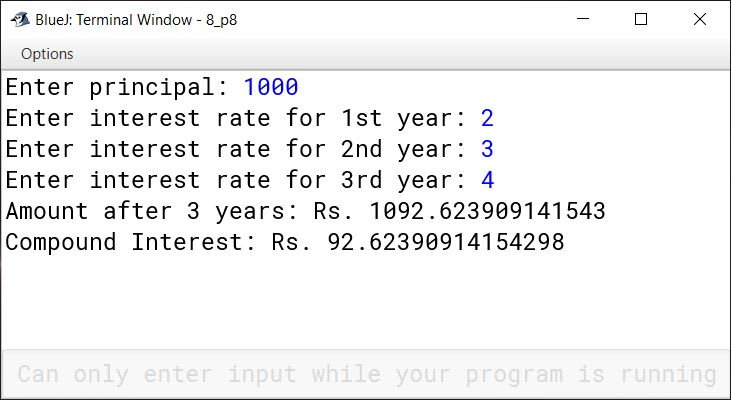# Mathematical Library Methods

## Multiple Choice Questions

#### Question 1

Given: double a = -8.35;
double p = Math.abs(Math.floor(a));
What will be the final value stored in the variable p?

1. 9.0
2. 8.0
3. 7.0
4. 8.5

9.0

Reason — Math.floor() method returns the largest double value that is less than or equal to the argument and is equal to a mathematical integer. As -9.0 is the largest mathematical integer less than -8.35 so it is returned in this case. Math.abs() returns the absolute value of its argument so the final output is 9.0. The expression is solved as follows:

p = Math.abs(Math.floor(a));
⇒ p = Math.abs(Math.floor(-8.35));
⇒ p = Math.abs(-9.0);
⇒ p = 9.0

#### Question 2

Given: double m = Math.max(Math.ceil(14.55),15.5);
What will be the final value stored in the variable m?

1. 15.0
2. 14.0
3. 15.5
4. 14.5

15.5

Reason — ceil() returns the whole number greater than or equal to the number and max() returns the greater number between its arguments. So, the expression is solved as follows:

m = Math.max(Math.ceil(14.55),15.5);
⇒ m = Math.max(15.0,15.5);
⇒ m = 15.5

#### Question 3

Given: d = Math.min(-15.5, -19.5);
What data type will you refer for the variable d?

1. int
2. float
3. double
4. None

double

Reason — The return data type of Math.min() function depends on its arguments. Here, the arguments are of double data type hence, Math.min() will return a double value. So, data type of variable d will be double.

#### Question 4

Given: double k = Math.rint(-9.4) + Math.sqrt(9.0);
What will be the final value stored in the variable k?

1. -5.0
2. -6.0
3. -6.4
4. -5.4

-6.0

Reason — Math.rint(-9.4) will give -9.0 as it is nearest integer to -9.4. Math.sqrt(9.0) will give 3.0. So, the expression is solved as follows:

k = Math.rint(-9.4) + Math.sqrt(9.0);
⇒ k = -9.0 + 3.0
⇒ k = -6.0

#### Question 5

Given double b = Math.ceil(3.4) + Math.pow(2,3);
What will be the final value stored in the variable b?

1. 12.0
2. 11.0
3. 11.4
4. 11.5

12.0

Reason — Math.ceil() returns the smallest whole number greater than or equal to its argument. Math.pow(a,b) returns the value ab. So, the final value of b will be:

b = Math.ceil(3.4) + Math.pow(2,3);
⇒ b = 4.0 + 8.0
⇒ b = 12.0

## State True or False

#### Question 1

The return data type of Math.log() is double.
True

#### Question 2

Java.Math class is used for different mathematical functions.
True

#### Question 3

The output of Math.abs(-99.99) is 100.00.
False

#### Question 4

Math.sqrt(-225) can't be defined.
True

#### Question 5

The output of Math.cbrt(-3) will be -27.
False

#### Question 6

Math.round() returns the value in float data type.
False

## Write down the syntax for the following functions

#### Question 1

To find the natural log of q.

Math.log(q)

#### Question 2

To find the cube root of a number m.

Math.cbrt(m)

#### Question 3

To find the round-off of a number k.

Math.round(k)

#### Question 4

To find the absolute value of a number y.

Math.abs(y)

#### Question 5

To find the exponent of a number p.

Math.exp(p)

## Explain the following functions

#### Question 1

Math.random()

It returns a positive double value, greater than or equal to 0.0 and less than 1.0.

#### Question 2

Math.pow()

It returns the value of the first argument raised to the power of the second argument. Data type of the two arguments and the return type of this method is double.

#### Question 3

Math.cbrt()

It returns the cube root of its argument as a double value.

#### Question 4

Math.log()

It returns the natural logarithm of its argument. Both return type and argument is of double data type.

## Distinguish between

#### Question 1

Math.ceil() and Math.floor()

Math.ceil( )Math.floor( )
It returns the smallest double value that is greater than or equal to the argument and is equal to a mathematical integer.It returns the largest double value that is less than or equal to the argument and is equal to a mathematical integer.
double a = Math.ceil(65.5);
In this example, a will be assigned the value of 66.0 as it is the smallest integer greater than 65.5.
double b = Math.floor(65.5);
In this example, b will be assigned the value of 65.0 as it is the largest integer smaller than 65.5.

#### Question 2

Math.rint() and Math.round()

Math.rint( )Math.round( )
It rounds off its argument to the nearest mathematical integer and returns its value as a double type.It rounds off its argument to the nearest mathematical integer and returns its value as an int or long type. If argument is float, return type is int, if argument is double, return type is long.
At mid-point, it returns the integer that is evenAt mid-point, it returns the higher integer.
double a = Math.rint(1.5);
double b =Math.rint(2.5);
Both, a and b will have a value of 2.0
long a = Math.round(1.5);
long b = Math.round(2.5);
a will have a value of 2 and b will have a value of 3

## Solutions to Unsolved Java Programs

#### Question 1

Write a program to calculate the value of the given expression:
1/a2 + 2/b2 + 3/c2
Take the values of a, b and c as input from the console. Finally, display the result of the expression to its nearest whole number.

```import java.util.Scanner;

public class KboatExpression
{
public static void main(String args[]) {

Scanner in = new Scanner(System.in);

System.out.print("Enter the value of a: ");
int a = in.nextInt();

System.out.print("Enter the value of b: ");
int b = in.nextInt();

System.out.print("Enter the value of c: ");
int c = in.nextInt();

double exp = (1 / Math.pow(a, 2)) + (2 / Math.pow(b, 2)) + (3 / Math.pow(c, 2));
long roundedExp = Math.round(exp);

System.out.println("Result rounded to whole number: " + roundedExp);
}
}```
##### Output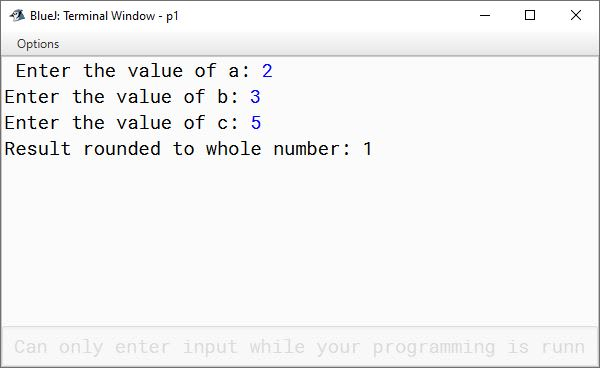#### Question 2

For every natural number m>1; 2m, m2-1 and m2+1 form a Pythagorean triplet. Write a program to input the value of 'm' through console to display a 'Pythagorean Triplet'.
Sample Input: 3
Then 2m=6, m2-1=8 and m2+1=10
Thus 6, 8, 10 form a 'Pythagorean Triplet'.

```import java.util.Scanner;

public class KboatPythagoreanTriplet
{
public static void main(String args[]) {

Scanner in = new Scanner(System.in);

System.out.print("Enter the value of m: ");
int m = in.nextInt();

if (m < 2) {
System.out.println("Invalid Input, m should be greater than 1");
return;
}

int firstTriplet = 2 * m;
int secondTriplet = (int)(Math.pow(m, 2) - 1);
int thirdTriplet = (int)(Math.pow(m, 2) + 1);

System.out.println("Pythagorean Triplets: "
+ firstTriplet
+ ", "
+ secondTriplet
+ ", "
+ thirdTriplet);

}
}```
##### Output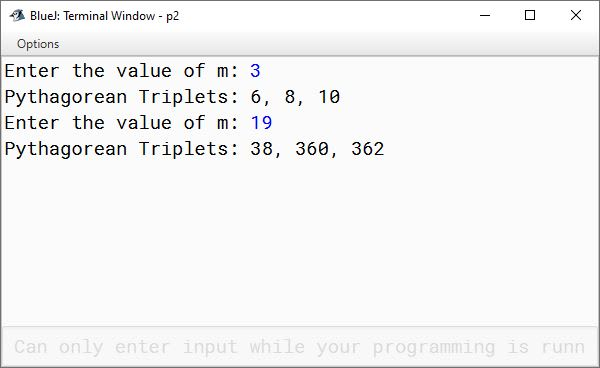#### Question 3

Write a program to input a number. Calculate its square root and cube root. Finally, display the result by rounding it off.
Sample Input: 5
Square root of 5= 2.2360679
Rounded form= 2
Cube root of 5 = 1.7099759
Rounded form= 2

```import java.util.Scanner;

public class KboatRoots
{
public static void main(String args[]) {

Scanner in = new Scanner(System.in);

System.out.print("Enter the number: ");
int num = in.nextInt();

double sqrtValue = Math.sqrt(num);
double cbrtValue = Math.cbrt(num);
long roundSqrt = Math.round(sqrtValue);
long roundCbrt = Math.round(cbrtValue);

System.out.println("Square root of " + num + " = " + sqrtValue);
System.out.println("Rounded form = " + roundSqrt);
System.out.println("Cube root of " + num + " = " + cbrtValue);
System.out.println("Rounded form = " + roundCbrt);
}
}```
##### Output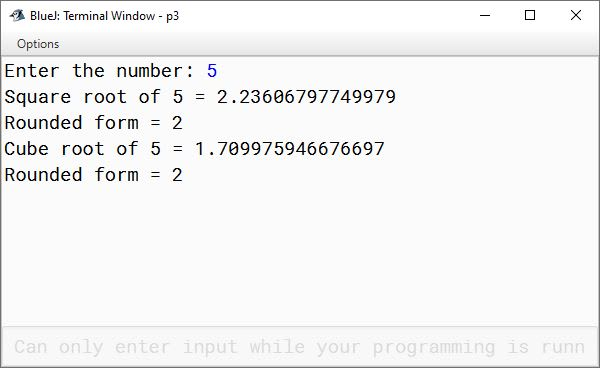#### Question 4

The volume of a sphere is calculated by using formula:
v = (4/3)*(22/7)*r3
Write a program to calculate the radius of a sphere by taking its volume as an input.
Hint: radius = ∛(volume * (3/4) * (7/22))

```import java.util.Scanner;

public class KboatSphere
{
public static void main(String args[]) {

Scanner in = new Scanner(System.in);

System.out.print("Enter volume of sphere: ");
double v = in.nextDouble();

double r = Math.cbrt(v * (3 / 4.0) * (7 / 22.0));
System.out.println("Radius of sphere = " + r);
}
}```
##### Output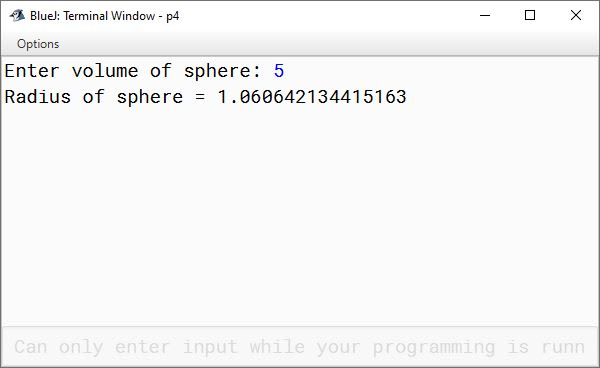#### Question 5

A trigonometrical expression is given as:
(Tan A - Tan B) / (1 + Tan A * Tan B)
Write a program to calculate the value of the given expression by taking the values of angles A and B (in degrees) as input.
Hint: radian= (22 / (7 * 180)) * degree

```import java.util.Scanner;

public class KboatTrigExp
{
public static void main(String args[]) {

Scanner in = new Scanner(System.in);

System.out.print("Enter angle A in degrees: ");

System.out.print("Enter angle B in degrees: ");
double angleBDeg = in.nextDouble();

double angleBRad = (22 * angleBDeg) / (7 * 180);

double trigExp = numerator / demoninator;

System.out.println("tan(A - B) = " + trigExp);
}
}```
##### Output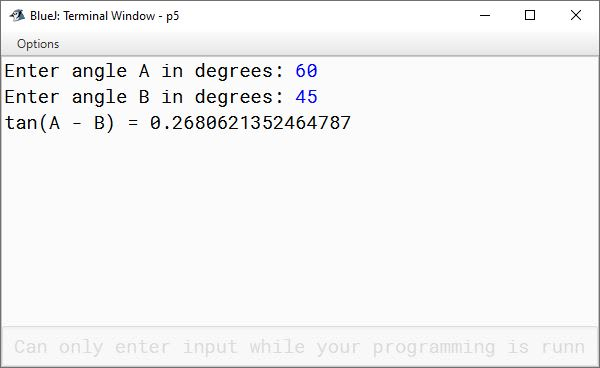#### Question 6

The standard form of quadratic equation is represented as:
ax2 + bx + c = 0
where d= b2 - 4ac, known as 'Discriminant' of the equation.
Write a program to input the values of a, b and c. Calculate the value of discriminant and display the output to the nearest whole number.

```import java.util.Scanner;

{
public static void main(String args[]) {

Scanner in = new Scanner(System.in);

System.out.print("Enter a: ");
int a = in.nextInt();

System.out.print("Enter b: ");
int b = in.nextInt();

System.out.print("Enter c: ");
int c = in.nextInt();

double d = Math.pow(b, 2) - (4 * a * c);
long roundedD = Math.round(d);

System.out.println("Discriminant to the nearest whole number = " + roundedD);
}

}```
##### Output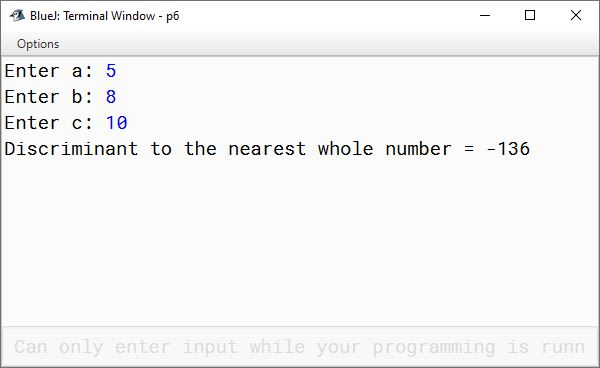#### Question 7

The sum of first n odd natural numbers can be calculated as n2, where n is the number of odd natural.
For example,
Sum = 1 + 3 + 5 + 7; number of odd natural = 4
Therefore, Sum = 42 = 16
Write a program to input number of odd natural terms and display sum of the given series:
(a) 1 + 3 + 5 + ................... + 29 + 31
(b) 1 + 3 + 5 + 7 + ................... + 47 + 49

```import java.util.*;

public class KboatCalculateSum
{

public static void main(String args[])
{
Scanner in = new Scanner(System.in);
System.out.print("Enter the number of odd natural terms: ");
int num = in.nextInt();

System.out.println("Number of odd natural terms = " + num);

double sum = Math.pow(num,2);
System.out.println("Sum = " + sum);

}
}```
##### Output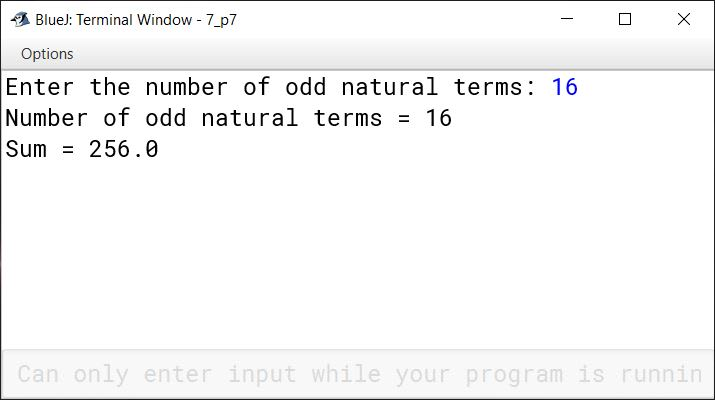#### Question 8

Write a program to input the sum. Calculate and display the compound interest in 3 years, when the rates for the successive years are r1%, r2% and r3% respectively.

Hint: [A = P * (1 + (r1 / 100)) * (1 + (r2 / 100)) * (1 + (r3 / 100)) and CI = A - P ]

```import java.util.*;

public class KboatCompoundInterest
{
public static void main(String args[])
{
Scanner in = new Scanner(System.in);

System.out.print("Enter principal: ");
double p = in.nextDouble();

System.out.print("Enter interest rate for 1st year: ");
float r1 = in.nextFloat();
System.out.print("Enter interest rate for 2nd year: ");
float r2 = in.nextFloat();
System.out.print("Enter interest rate for 3rd year: ");
float r3 = in.nextFloat();

double amt = p * (1 + (r1 / 100))
* (1 + (r2 / 100))
* (1 + (r3 / 100));
double ci = amt - p;

System.out.println("Amount after 3 years: Rs. " + amt);
System.out.println("Compound Interest: Rs. " + ci);
}
}```
##### Output